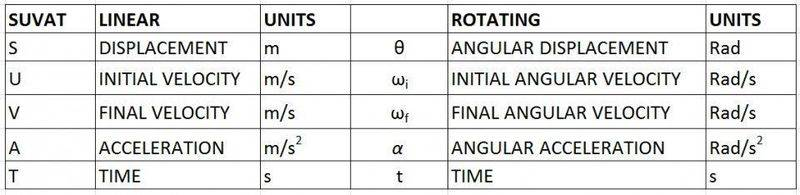# Angular Speed and Kinetic energy

• deathkamp

## Homework Statement

Ok I have the answers to parts a and b, which I am 100% confident in since they are simple computations
Question is in attachement

My issue comes in when I have to get parts c
The mass of the cylinder is 8.7kg,
its diameter is .18m,
its .35m long and
angular acceleration of 33.2m/s^2

**note please ignore the markings on the image.

w=theta/t
w=v/r

## The Attempt at a Solution

for part c) angular speed=angular velocity, w=theta/t
I am not sure about how to get the angular speed at 35 radians

#### Attachments

•angular speed an kinetic.png
44.4 KB · Views: 530
Last edited:
My issue comes in when I have to get parts c and d. The angular speed=angular velocity, formula wise so I get 35rad/2.25s so my part is

It's not correct. Please show your working. What formula did you use?

part d) since its rotation by 35 radians, KE=(1/2)*i*w^2 where i is inertia, and its i=S*r^2*d*m, S=r*theta
S=315, i=4000 rounded, KE=149.237J, my w=74.7 which I got from part a

i is the "moment of inertia" not just "inertia". Check the equation for the moment of inertia of a cylinder. I don't recognise i=S*r^2*d*m where S=r*theta

For part c I did 35rad/2.25s=15.5, since angular speed =angular velocity wouldn't they have the same formula? Unless there is an angular speed equation that I am not aware of?
And for part D Ill hold off on it for now, since I edited my post an already deleted it.

I'm off to bed as soon as I post this because it's after midnight here.
For part c I did 35rad/2.25s=15.5, since angular speed =angular velocity wouldn't they have the same formula?

That would be correct if it was rotating at a constant angular velocity but it's not, it's accelerating.

If a car accelerated from rest and covered 100m in 10 seconds it's final velocity wouldn't be 10m/s. That would be the average. It would be slower at the start and faster at the finish. You would use the SUVAT equations to find the final velocity.

Back tomorrow.

For part b all I did was multiply the angular velocity by the time to get the Angle in radians. so 74.7*2.25=168.08rad

I am getting 48.2rad/s for part c, which SUVAT formula did you use? Please tell me?

And for part D I found out that since the object can rotate or accelerating(translating) in a linear direction(PLEASE READ THE PROBLEM BACK CAUSE IM NOT SURE IF ITS BOTH, just to confirm with me) I need to add the kinetic energy of
KElinear=(1/2)*m*v^2 and KErotate=(1/2)*i*w^2
where i is the inertia, an its equal to i=(1/2)*m*r^2 because its a solid cylinder I checked equation online
V is Vcylinder=√((4/3)*g*h) and
w=v/r.

For v=(√((4/3)* 9.8* .35))=2.138
KElinear=.5*(8.7)*(2.138^2)=19.88joules

For r= .09m converted cm to m i=.5* 8.7* (.09^2)=.035 w=(2.138/.09)=23.7
KErotate=.5* .035* (23.7^2)=9.82Joules

So in summary, I need to know what formula you used for part c, then I would like to confirm if this entire problem has just rotational kinetic energy or both, rotational and translation, and if I missed anything please let me know. I won't be able to respond when you wake up because you are 5 hours ahead of me, so please put in as much info as you can cause I need to master this problem before I go into my class, Thank you.

Last edited:
Sorry for double post, but did you get around to look at this CWatters?

For part b all I did was multiply the angular velocity by the time to get the Angle in radians. so 74.7*2.25=168.08rad
The velocity isn't constant so that doesn't work. Have another go using the equations for motion under constant acceleration.
I am getting 48.2rad/s for part c, which SUVAT formula did you use? Please tell me?
I got the same answer. Forum rules don't allow us to just give the answers or do too much of the work for you. If you show me your working I can give hints and point out where you have made a mistake if any.
And for part D I found out that since the object can rotate or accelerating(translating) in a linear direction(PLEASE READ THE PROBLEM BACK CAUSE IM NOT SURE IF ITS BOTH, just to confirm with me)
The problem mentions a flywheel. These normally rotate on a fixed axis. So I think this problem only involves rotation not translation.
... KErotate=(1/2)*i*w^2 where i is the inertia, an its equal to i=(1/2)*m*r^2 because its a solid cylinder I checked equation online
Yes that is correct.
For r= .09m converted cm to m i=.5* 8.7* (.09^2)=.035
Yes that is correct
w=(2.138/.09)=23.7
KErotate=.5* .035* (23.7^2)=9.82Joules
That's not correct.
ω is the angular velocity. You calculated the angular velocity after 2.25 seconds as 74.9 rad/s in question a).

Useful reference (better version)..Last edited:
For part c, the angular speed is an inital or final speed? meaning is it w0 or w?

For part c, the angular speed is an inital or final speed? meaning is it w0 or w?

The question asks for the angular velocity after turning. So it is the final angular velocity you need to calculate.

I would use V = w0 + A*T correct?, ok Ived tried a few of them with V in them and none are giving me 48.2. I used, for example tried theta=((w0+v)/2)*t and V^2=w0^2+2*A*theta, can you please tell me what I am doing wrong?

Last edited:
Sorry I had to delete a few posts. I'm rushing too much. The equation you need is..

ωf2 = ωi2 + 2αθ
which is similar to
V2 = U2 + 2as

ωi = 0 (starts from rest)
α = 33.2 rad/s2 (from the problem)